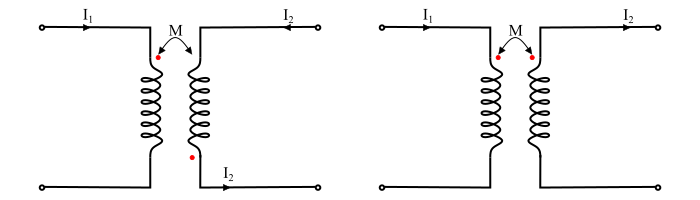# Mutual Inductance with Dot Convention

When the EMF is induced by self and mutual inductance occur simultaneously, the relative polarities of these two induced EMFs must be determined before making any circuit calculations. The induced EMF in a coil due to mutual inductance may either aid or oppose the induced EMF due to self-inductance depending upon the sense of the windings and the directions of the currents in the coils.

## Dot Notation or Dot Rule

The dot rule or dot convention may be stated as follows −

• If both currents enter the dotted ends of the coupled coils or if both currents enter undotted ends, then the sign of mutual inductance (M) will be same as the signs of the self-inductance (L).

• If one current enters a dotted end and the other enters at the un-dotted end, the sign of the mutual inductance (M) will be opposite to that of the self-inductance (L).

## Explanation

If the two currents flow into each dot or out of each dot, the mutual inductance (M) will be positive. On the other hand, if one current flows into a dotted end and the other leaves the dotted end, then the mutual inductance (M) will be negative.

Consider the following circuit examples −

• The mutual inductance (M) is positive because both the currents $I_{1}$ and $I_{2}$ flow into dots.• The M is positive because both the currents $I_{1}$ and $I_{2}$ flow out of the dots.• The M is negative since the current $I_{1}$ flows into the dot whereas the current $I_{2}$ flows out of the dot.## Circuit Equations for Coupled Inductors

The following circuit examples illustrate the writing of circuit equations for the coupled circuits.

• Circuit 1

Consider a coupled circuit consisting of two inductors $L_{1}$ and $L_{2}$. Let $V_{1}$ and $V_{2}$ are the voltages being applied to respective inductors. Each of the two currents $I_{1}$ and $I_{2}$ are assumed to enter the dotted ends. Therefore, the sign of the mutual inductance (M) being positive.• By applying KVL, the voltage equations can be written as,

$$\mathrm{V_{1}=L_{1}\frac{di_{1}}{dt}+M\frac{di_{2}}{dt}\:\:\:\:\:\:...(1)}$$

$$\mathrm{V_{2}=L_{2}\frac{di_{2}}{dt}+M\frac{di_{1}}{dt}\:\:\:\:\:\:...(2)}$$

• Circuit 2

Consider a coupled circuit consisting of two inductors $L_{1}$ and $L_{2}$. Let $V_{1}$ and $V_{2}$ are the voltages being applied to respective inductors. The current $I_{1}$ is assumed to enter the dotted terminal whereas the current $I_{2}$ is leaving the dotted terminal. Therefore, the sign of the mutual inductance (M) being negative.